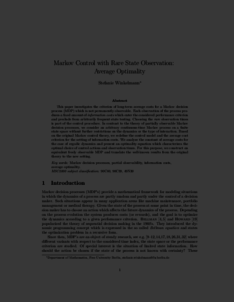Repository: Freie Universität Berlin, Math Department

# Markov Control with Rare State Observation: Average Optimality

Winkelmann, S. (2017) Markov Control with Rare State Observation: Average Optimality. Markov Processes and Related Fields, 23 . pp. 1-34. ISSN 1024-2953Preview

681kB

Official URL: http://math-mprf.org/journal/articles/id1446/

## Abstract

This paper investigates the criterion of long-term average costs for a Markov decision process (MDP) which is not permanently observable. Each observation of the process produces a fixed amount of information costs which enter the considered performance criterion and preclude from arbitrarily frequent state testing. Choosing the rare observation times is part of the control procedure. In contrast to the theory of partially observable Markov decision processes, we consider an arbitrary continuous-time Markov process on a finite state space without further restrictions on the dynamics or the type of interaction. Based on the original Markov control theory, we redefine the control model and the average cost criterion for the setting of information costs. We analyze the constant of average costs for the case of ergodic dynamics and present an optimality equation which characterizes the optimal choice of control actions and observation times. For this purpose, we construct an equivalent freely observable MDP and translate the well-known results from the original theory to the new setting.

Item Type: Article Markov decision processes, partial observability, information costs, average optimality. Mathematical and Computer Sciences > Mathematics > Applied Mathematics Department of Mathematics and Computer Science > Institute of Mathematics > BioComputing Group 1965 Ulrike Eickers 04 Oct 2016 14:01 29 Jun 2017 09:36

Repository Staff Only: item control page